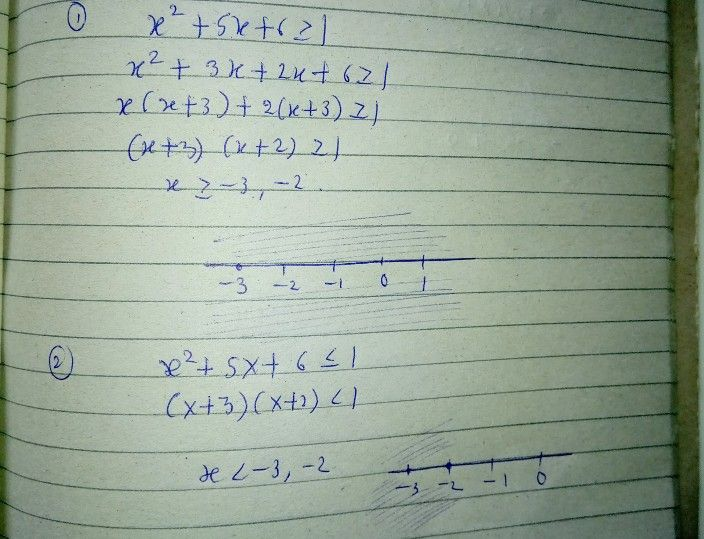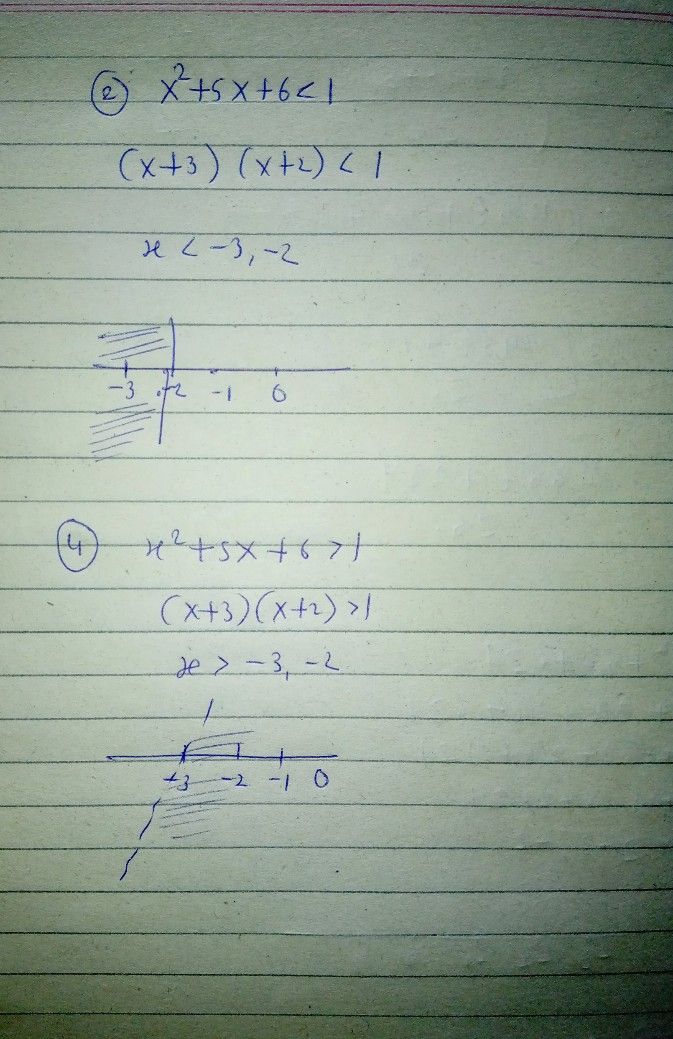Symbol
ProblemActivity $2$ $0irection$ Solve the following inequalities. Sketch the graphs of each inequalities $0n$ $a$ number line. $1.x^{2}+5x+6\geq 1$ $3.x^{2}+5x+6\leq 1$ $2$ $x^{2}+5x+6<1$ $4$ $x^{2}+5x+6>1$
10th-13th grade
Calculus
Search count: 106
SolutionQanda teacher - RASHMIKAany doubt so pls ask me to know
kindly evaluate ans with gift coins if you are satisfied with my ans
thanks
best of luckStudent
thank you?